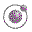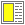The Order of Evaluation - slide 18 : 26

Conditionals and sequential boolean operators
There are functional language constructs - special forms - for which applicative order reduction would not make sense
• (if b x y)

• Depending on the value of b, either x or y are evaluated

• It would often be harmful to evaluate both x and y before the selection

• (define (fak n) (if (= n 0) 1 (* n (fak (- n 1)))))

• (and x y z)

• and evaluates its parameter from left to right

• In case x is false, there is no need to evaluate y and z

• Often, it would be harmful to evaluate y and z

• (and (not (= y 0)) (even? (quotient x y)))